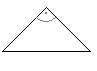The Compendium Geometry is an eBook providing facts, formulas and explanations about geometry.## Triangle - General DefinitionsA triangle is a polygon which consists of three sides (sometimes also called a trigon). Normally the vertices of the triangle are labeled counterclockwise by the uppercase letters A, B, and C. Each vertex has a vertex angle which is labeled by the corresponding greek letter, α, β, γ, respectively. The sides of the triangle are labeled by lowercase letters, corresponding to the particular opposite vertex.

There are several special triangles: acute triangle, obtuse triangle, equilateral triangle, isosceles triangle, right triangle, and isosceles right triangle.

 Type of triangle Properties acuteA triangle is said to be acute if all three angles are acute (i.e. have values less than 90°). obtuseA triangle having an angle α which is greater than 90° is said to be obtuse. The other two angles are necessarily acute. equilateralAn equilateral triangle is a triangle with all sides being equal. isoscelesIf two sides of a triangle are equal but different to the third side, this triangle is called an isosceles triangle. rightA right triangle has one angle with exactly 90°. The sides of a right triangle have special names: the side opposite the right angle is called hypothenuse, the other two are called legs. isosceles rightAn isosceles triangle having an angle of 90° is called an isosceles right triangle.

Last Update: 2011-01-11